## string simulator physics and mathematics

by Theo Verelst
(Last update Oct 6, 2006)

### Introduction

I've made a string simulator which is a program running on a fast PC or unix workstation to simulate the motion of strings in a musical instrument, based on a physical model of a vibrating string. A string like on a guitar or even in a piano is suspended between two fixed points, which are to begin with thought to be unmoving, and is though to be brought into a natural motion by displacing it, thus straining it, and then releasing the virtual string, computing how it will move.

From the computed motion, a sound can be derived, which is made audible through a computer sound device. To make the sound more interesting and the motion more complex to understand and predict, more than one string can be sounded at the same time and coupled to eachother.

See also the program oriented page about the actual software computing the simulaneous motion of up to 10 strings (on a very fast machine), of which a live demonstration has been presented at FOSDEM conference at brussels university, the demonstration audio files and open source software files are on the web.

### The suspended string

A string in this case is considered to be a thin stretch of metal which is under tension and with fixed supports on both ends, and which is free to move, to begin with only in two dimensions, such that excursion from the rest condition (where the string is without counting gravity a straight line) is a scalar, not a vector.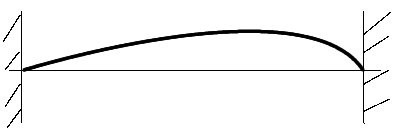A string in motion, suspended on fixed points at its ends.

The string has a finite weigth and a known length and moves after is is exited by striking it or plucking it, or in mathematical physical sense giving it an initial state unequal to the rest state, and is assumed to move according to the newtonian and basic equations of motion.

Because there are no easy closed form solutions to the evolution of the string state starting from a random initial state, I use an approximation, a model of the problem, where the string is like in finite element analysis cut up in pieces (order of magnitude: 10^2) of equal weight which all follow the 2d order equtions of motion, preferably inintegral form, and connect together over elastically behaving string segments.

### A single harmonic oscillator as string section

Consider one of the harmonic oscillator sections of the cut-up string: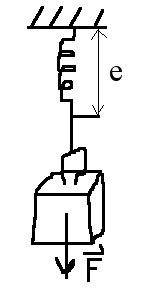The Harmonic swing with excursion e. Gravity would excert a force F = g . M  , where M is the mass of the  weight, just like the spring will excert a force in the other direction on the weight, depending on how much the spring is chaged, proportional to the excursion e.

Commonly known this system will oscillate as a perfect sine wave when exited and when there is no friction or interaction with other systems.

The piece of string weight in this case is hung from a fixed mass, the ceiling or so, which doesn't move or influence the oscillation.

### The string as coupled harmonic oscillators

To simulation the motion of the whole string, a little piece of metal from the string is considered to represent a fraction of the weigth of the string and is considered connected to the adjacent pieces of weight of the string and the string length is taken to be madeof an equal number of little springs, which connect the weight fractions together: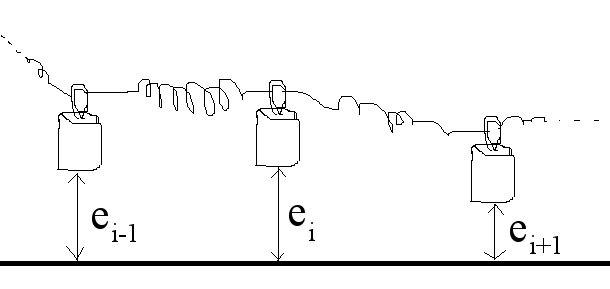so as to form a set of connected or coupled harmonic oscillators with floating suspension points.

At any point along the string, there is a force acting upon the weight concentrated in that point which is the sum of the two forces from the neighbouring points, which are the products of the string constants and the displacement differences, including the signs.

For each mass concentration point, all with equal weights, the well known Newtonian equations of motion hold, the velocity is the integral of the force acting on the particle per time interval, the displacement is the integral of the velocity at any point in time. And to make the local harmonic oscillator work, the springs excert a force proprtional and opposite to the displacement with respect to local equilibrium.

For a small  integration approximation step size, one could take a linearisation, and perform simple additions to compute the integrals of velocity and displacement.

To do this integration approximation over all the string segments aka mass concentration points, a sweep over all segments is done in a fixed direction over all the points. Since accuracy is a big problem, because a hundred coupled harmonic oscillatlors generate a lot of total rounding noise, the practical real-time simulation uses a normalisation which requires no multiplications, so no rounding errors of the rational kind are made at each sweep step.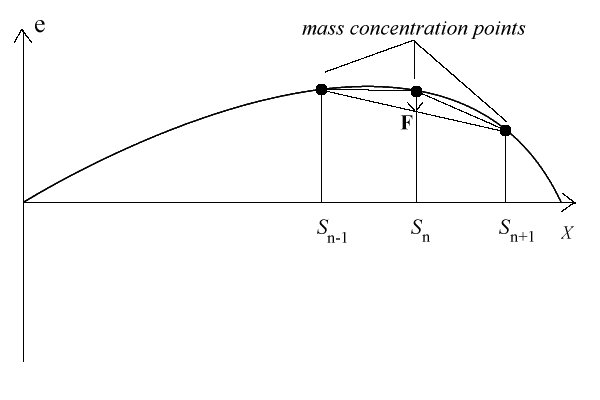### String damping, lossyness

A natural string always experiences damping, both from air friction and from internal friction in the string and at the end points, related to the clamping of the material and the suspension point material. As a result the sound of the string decays with time, and also the tonal quality changed with time, and by and large gets duller after a while.

To make the string damped a bit, so that it sounds softer (and different) after a while, the end points are considered a bit stiff and a bit damped. This in the practical simulation is done by forcing the excursion down around the end points, gradually increasing the forcedown toward complete damping at the two string suspension points.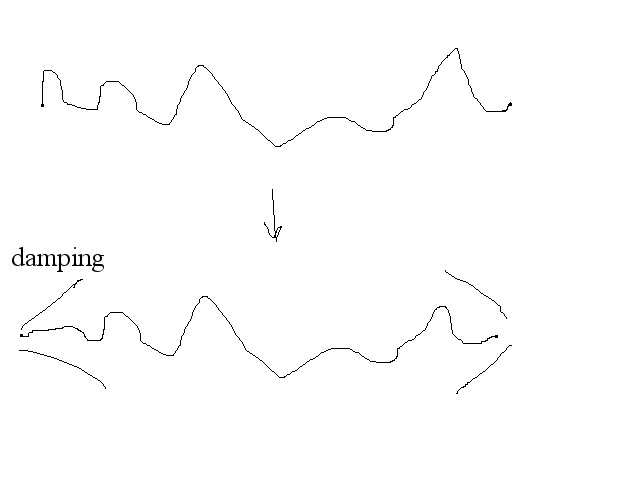This has an effect on the energy in the string as a whole such that after a while the motion of the string overall decreases in amplitude, it makes the virtual string length dependent on the frequency components which are in it (which is known also to happen in piano strings), and probably introduces a certain type of (unwanted) linear distortion to the simulation.

As a result, the string behaves a bit like a normally suspended string which is also a bit stiff close to the end points, though not necessarily contractive.

### More issues taken into account in the simulator: coupling, non-linear amplification, feedback

Two more extensions are added to get a good sounding simulation string instrument, apart from real-time parameter changes; string coupling, and a overall feedback with non-linear characteristics.

Of course a challenge for this problem which is different from other simulations is to get a decaying string sound which is rendered as a sound file over a long simulation interval, for instance a number of seconds. At CD sample rate, that means hundreds of thousands of simulation steps, and a noise preferably less then 2^-15 (is less then 0.004 % of maximum amplitude) top to top amplitude.

The simulation software in its various versions also allows research related work by outputting (also in interaction time) string excursion and velocity and drawing graphs of these using Tcl/Tk scripts connected over a socket.

And a real time simulation requires serious computer horsepower, even when programmed very efficiently in C.

### The quality of the simulation and sound properties

It is possible to use a reflection formula to estimate string motion, and of course helmholz like standing wave approaches.

In general, the qualities the author was looking for are lost in many ways by doing so. A lot of the wonderfullness of a sounding string comes from all the complexities of the physical string and its interaction with an enclosure and with other strings during the sounding of a musical chord.

Listen to the demo sounds to get an idea.